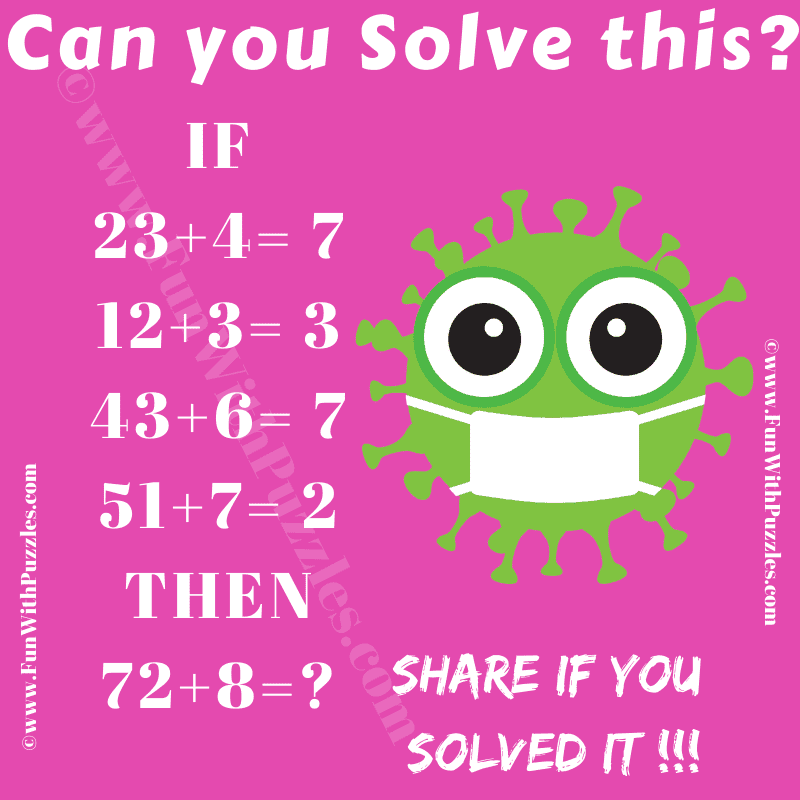Here are the logic puzzles with numbers that will twist your brain. In each of these maths logic puzzles, you are shown some logical equations. Your challenge in these puzzles is to find the hidden logic in these equations which makes these equations logical correct. Once you are able to find the hidden logic, find the value of the missing number in the last equation.1. Can You Find the Missing Number?

The answers to these "logic puzzles", can be viewed by clicking on the answer button.